International
Tables for
Crystallography
Volume F
Crystallography of biological macromolecules
Edited by M. G. Rossmann and E. Arnold

International Tables for Crystallography (2006). Vol. F, ch. 14.1, p. 296   | 1 | 2 |

## Section 14.1.8. The phase probability distribution for anomalous scattering

B. W. Matthewsa*

aInstitute of Molecular Biology, Howard Hughes Medical Institute and Department of Physics, University of Oregon, Eugene, OR 97403, USA
Correspondence e-mail: brian@uoxray.uoregon.edu

### 14.1.8. The phase probability distribution for anomalous scattering

| top | pdf |

From Fig. 14.1.8.1, it can be seen that the most probable phase angle will be the one for which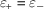. At any other phase angle, there will be an `anomalous-scattering lack of closure' which we define to be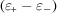. The value ofcan readily be calculated as a function of ϕ (Matthews, 1966b; Hendrickson, 1979). Thus, if the r.m.s. error inis, and the distribution of error is assumed to be Gaussian, then from measurements of anomalous scattering, the probability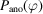of phase ϕ being the true phase ofcan be estimated using an equation exactly analogous to equation (14.1.4.2).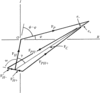Figure 14.1.8.1 | top | pdf |Vector diagrams illustrating lack of closure in the anomalous-scattering method.

An example of an anomalous-scattering phase probability distribution is shown by the dotted curve in Fig. 14.1.8.2. The asymmetry of the distribution arises from the fact thatis the phase probability distribution forrather than that of, which would be symmetrical about the phase of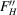. The overall probability distribution obtained by combining the anomalous-scattering data with the previous isomorphous-replacement data (Fig. 14.1.2.1b) is given by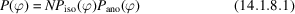and is illustrated in Fig. 14.1.8.2.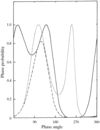Figure 14.1.8.2 | top | pdf |Combination of isomorphous replacement and anomalous-scattering phase probabilities for a single isomorphous replacement.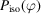is drawn as a solid line,as a dotted line, and the combined probability distribution is drawn as a dotted-and-dashed line.

### References

Hendrickson, W. A. (1979). Phase information from anomalous-scattering measurements. Acta Cryst. A35, 245–247.Google Scholar
Matthews, B. W. (1966b). The extension of the isomorphous replacement method to include anomalous scattering measurements. Acta Cryst. 20, 82–86.Google Scholar